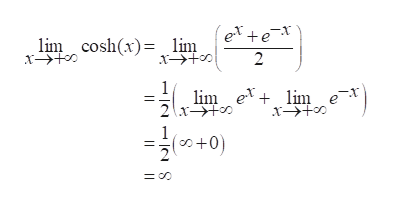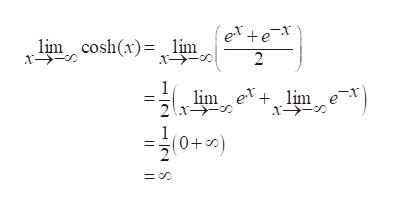The hyperbolic cosine function, denoted cosh x, is used to model the shape of a hanging cable (a telephone wire, for example).  It is defined as cosh x = e^x + e^-x / 2. a. Determine its end behavior by evlauating the limit of cosh x from x from positive inifinity and the limit of cosh x from x from negative inifnity.b. Evaluate cosh 0.cosh 0 = Use symmetry and part (a) to sketch a plausible graph for y = cosh x.

Question

The hyperbolic cosine function, denoted cosh x, is used to model the shape of a hanging cable (a telephone wire, for example).  It is defined as cosh x = e^x + e^-x / 2.

a. Determine its end behavior by evlauating the limit of cosh x from x from positive inifinity and the limit of cosh x from x from negative inifnity.

b. Evaluate cosh 0.

cosh 0 =

Use symmetry and part (a) to sketch a plausible graph for y = cosh x.

Step 1

Given information:

Tyhe given function is cosh(x).

Step 2

Calculate:

First, evaluate the limit of cosh x from x from positive inifinity and then the limit of cosh x from x from negative inifnity.help_outlineImage Transcriptionclose(ex+ex 2 lim cosh(x) lim x 1 lim e lim e 1 (+0 fullscreen
Step 3help_outlineImage Transcriptioncloseex+e- 2 lim coshx)lim lim ex+ lim e (+0) fullscreen

Want to see the full answer?

See Solution

Want to see this answer and more?

Our solutions are written by experts, many with advanced degrees, and available 24/7

See Solution
Tagged in

Calculus